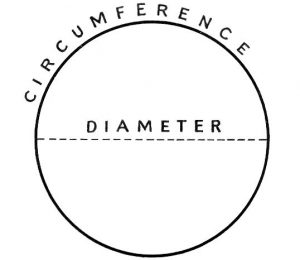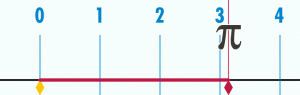Pi is somewhat of a magic number used in mathematics. It is defined as the ratio of the circumference of a circle to its diameter. The circumference is the distance around a circle, or its perimeter. The diameter is the line segment passing through a circle’s origin whose endpoints lie on its perimeter. Pi is an irrational number, meaning it cannot be written as a ratio of two integers. Pi has been calculated to 31.4 trillion digits—a calculation only made possible using computers!

####What is The History of Pi

Pi transcends cultures and centuries. In truth, math is a language and it’s one that can be understood across many different types of peoples. From the Babylonians and Egyptians, to Archimedes, and the Chinese, Indian, Arab, and European cultures, pi has been analyzed, refined, and used for 4000 years!

Records of Pi stretch as far back as 2000 BCE, when the Babylonians approximated it at 3.125. Ancient Egyptians approximated Pi at 3.16 in 1650 BCE. It wasn’t until Archimedes, a mathematician and inventor known for Archimedes’ principle, who arrived at our modern definition of pi, 22/7 or 3.14.The symbol for pi, π, was first used by British mathematician William Jones in the 1700s. Later popularized by a Swiss mathematician Leonhard Euler, it is considered one of the founders of pure mathematics. The symbol represents the ratio of pi, or 22/7.

#### What is The Importance of Pi

Pi is not the type of thing that you use once in school and soon forget. It’s found frequently in equations involving area, lengths of arcs, volumes, as well as in physical and engineering problems involving motion, vibration, and electricity. As an astronomy and physics student in college, I would have been lost if I didn’t know the value of pi – it shows up everywhere!

Area of a circle – A=πr^2

Fourier SeriesSurface Area of a Sphere – S=4πr^2

Volume of a Sphere – V=4/3 πr^3

#### Why Pi is Worth Celebrating

Pi Day is recognized as March 14, or 3.14, since the first three digits of pi are 3.14. In the classroom, Pi Day is a great way to introduce mathematical ratios and set the foundations for complex equations that will show up later in a student’s academic life. And maybe there will be a slice of pie involved – fingers crossed!As early as first grade, students are composing two-dimensional shapes like circles and partitioning these circles into equal shares. Throughout elementary school, students build on these ideas and transpose them to discuss ratios, areas, and more. By seventh grade, students know the formulas for area and circumference of a circle and understand how to solve for each. By the end of middle school, this knowledge is scaffolded into solving for the volumes of cones, cylinders, and spheres. In high school, students will need to understand and apply theorems about circles, using all the information they’ve previously learned.Circles are a focal point of K-12 geometry. It is reasonable to start pi celebrations with students of all age levels! If your school can get on board with each grade level celebrating Pi Day, then the students can do activities appropriate for their age level and build on what they did the previous Pi Day. For example, 1st graders can celebrate by drawing circles while 4th graders can recognize lines of symmetry in circles to identify the diameter. By making it a school-wide event, students will look forward to exploring the wonders of pi each year! Additionally, you’ll be building a deep knowledge around a topic that will follow them throughout their K-12 career into college and the STEM workforce.

If you need some ideas for how to celebrate Pi Day, check out these awesome, free resources on STEM Universe!